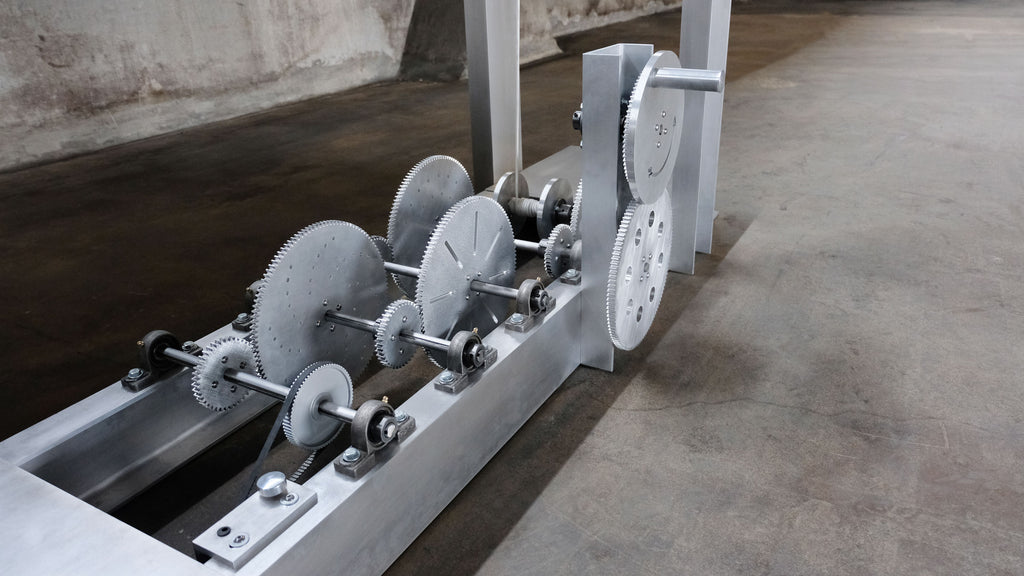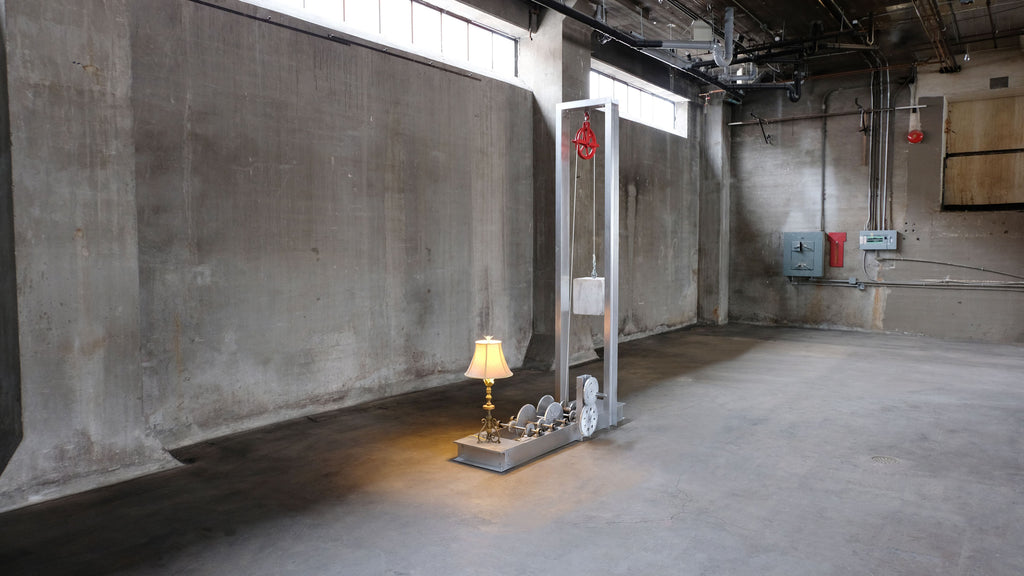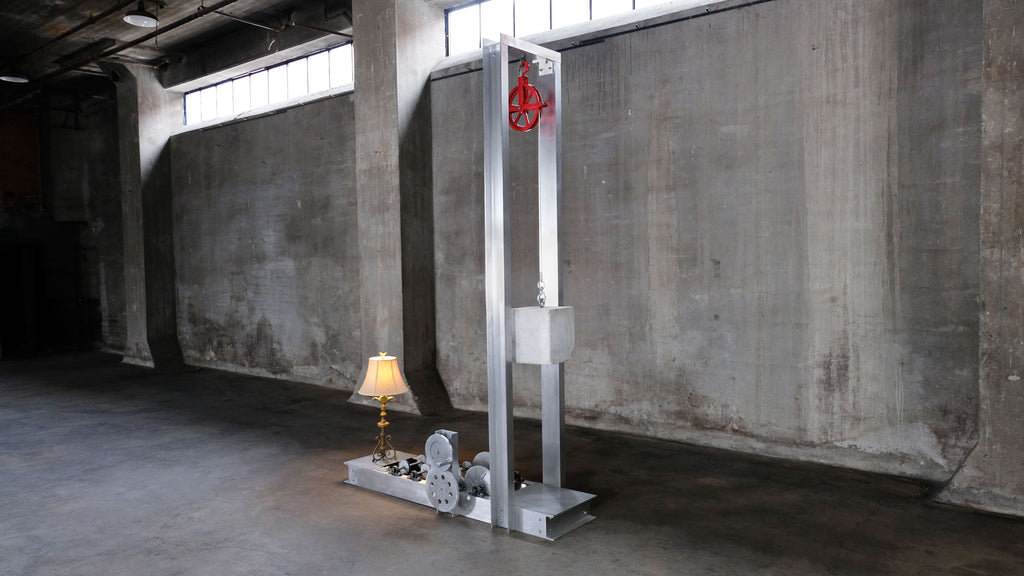# Ug = mgh

A “gravity battery” is a type of machine that creates and stores energy through raising a mass and allowing it to fall. This type of machine explores the Laws of Thermodynamics, the first of which describes how energy is not created or destroyed, but is transferred from one form into another form. Here, gravitational potential energy is transferred into kinetic energy, which is then transformed into light.

Ug= mgh illustrates these principles through the controlled fall of a 200 lb cube of marble, which is used to power LED lights on a table lamp. The marble block is suspended from a pulley 10’ high. Once the marble begins its descent, it will drive a custom-machined aluminum gear set, which turns a small dynamo motor that powers the table lamp. For every rotation of the first gear, the motor turns 146 times.

The title Ug= mgh describes the formula at work. The Ug in the equation refers to gravitational potential energy. When being lifted, the marble cube is gaining gravitational potential energy, since work is required to elevate the object against gravity. At its peak, the object possesses its maximum gravitational potential energy. Then mgh is the relationship between the object’s mass, its acceleration due to gravity, and the height it falls from. The gravitational potential energy is converted into kinetic energy as the mass is raised and then through its fall.

It takes the marble block roughly 10 to 14 minutes to fall completely, depending on the motor’s distance from the final gear. At which point, the weight will need to be cranked back up to the top again. Mechanical advantage of the crank allows the user to easily reset the machine.